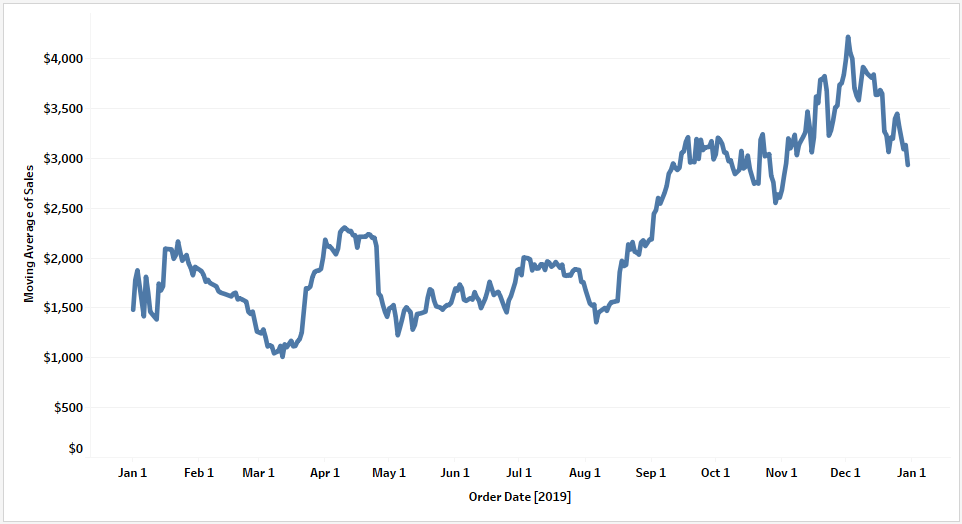top of page
Search
• Bernard K

# Tableau Moving Average

Updated: Nov 7, 2022In statistics, moving average is a calculation to analyze data points by creating a series of averages of different subset of the full data set. It is also called moving mean or rolling mean. This type of calculation is commonly used in time series data to smooth out short-time fluctuation – hence revealing the trend of data.

Given a series of data points, the first element of the moving average is obtained by taking the average of the initial fixed subsets of data points, then the subset is modified by shifting forward i.e. By excluding the first subset of data points and including the next subset of data points.

### How can I compute moving average in Tableau?

Using Superstores data set, you might be interested in looking at the overall trend of Sales. Without computing moving average, it is hard to tell what the trend of this data is. In the example below shows a dual chart – with one line (blue line) computing the moving average while the other line (orange line) returning the aggregates of each data point. The trend of this data is quite visible using the moving average unlike when using the aggregated data points (especially when dealing with lots of data points).### Let’s compute moving average to show the trend of Sales in 2019

• Drag dimension Order Date to the columns shelf – set as a continuous field showing exact date

• Drag SUM(Sales) to the rows shelf

• In this case have filtered data to show only year 2019 (optional)Right click on the dimension SUM(Sales) or open the drop-down menu >> Quick Table Calculation >> Moving AverageBy default, Tableau will compute the moving average using the previous two data points. However, you can customize your computation to meet your objective. To do so, open the drop-down menu >> Edit Table Calculation…On the pop-up dialogue box, customize your computation.

In this case, am computing moving average using the previous 30 data points from the current value.

Note: You can customize how your computation is executed using different options available in this menu.Executing this we’ve the view below which is a much better view in interpreting the trend of Sales in the year 2019.If you like the work we do and would like to work with us, drop us an email on our Contacts page and we’ll reach out!